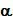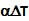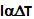## Monday, March 30, 2009

### Irodov Problem 1.290

Let the coefficient of linear thermal expansion be. When the temperature increases bythe length of the rod increases by a fraction. So if the length of the rod is l then the increase in length of the rod will be. In order for the length of the rod to be constant enough stress should be applied to compress the rod by. The strain will thus be. The stress (pressure) required will be.rupinder said...

sir, i have a doubt in Q.1.290

if the temp increases, then the natural length of the the cylinder increases. hence, the force applied would actually compress the cylinder to keep its length constant.
So, should'nt the required strain be
strain=
{L-L(1+E delta(t))}/L(1+E delta(t))?
E= coff. of expansion